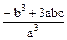# Mathematics

### Chapter : Polynomials

#### Symmetric Functions of zeros of a Quadratic Polynomial

Symmetric Function :
An algebraic expression in a and b, which remains unchanged, when a and b are interchanged is known as symmetric function in a and b.
For example, α2 + β2 and α3 + β3 etc. are symmetric functions.
Symmetric function is to be expressed in terms of (α + β) and αβ. So, this can be evaluated for a given quadratic equation.

Some useful relations involving α and β
1. α2 + β2 = (α + β)2 – 2αβ
2. (α – β)2 = (α + β)2 – 4αβ
3. α2β2 = (α + β) (α – β) = (α + β)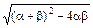4. α3 + β3 = (α + β)3 – 3αβ (α + β)
5. α3β3 = (α – β)3 + 3αβ (α – β)
6. α4β4= [(α + β)2 – 2αβ]2 –2(αβ)2
7. α4 – β4 = (α2 + β2) (α2 – β2) then use (1) and (3)

Example: If a and b are the zeroes of the polynomial ax2 + bx + c. Find the value of (i) α – β (ii) α2 – β2.

Solution: Since a and b are the zeroes or roots of the polynomial ax2 + bx + c.
⇒ a + b = –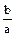; ab =(i) (a – b)2 = (a + b)2 – 4ab
== –=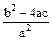a – b =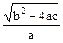(ii) a2 + b2 = a2 + b2 + 2ab – 2ab
= (a + b)2 – 2ab
=– 2=Example: If a and b are the zeroes or roots of the quadratic polynomial ax2 + bx + c. Find the value of (i) a2 – b2 (ii) a3 + b3.

Solution: Since a and b are the zeroes of ax2 + bx + c
⇒ a + b = –, ab =(i) a2 – b2 = (a + b) (a – b)
= –= –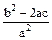= –= –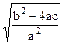(ii) a3 + b3 = (a + b) (a2 + b2 – ab)
= (a + b) [(a2 + b2 + 2ab) – 3ab]
= (a + b) [(a + b)2 – 3ab]
= –=–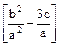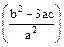=